# Abstracts

Bayesian approach; classical approach; degradation data analysis; reliability

Reliability assessment using degradation models: bayesian and classical approaches

Marta Afonso FreitasI, * * Corresponding author / autor para quem as correspondências devem ser encaminhadas ; Enrico Antonio ColosimoII; Thiago Rezende dos SantosII; Magda C. PiresII

IDep. de Eng. de Produção / Escola de Engenharia / Universidade Federal de Minas Gerais (UFMG) Belo Horizonte - MG;marta@dep.ufmg.br, marta.afreitas@gmail.com

IIDepartamento de Estatística / ICEX / Universidade Federal de Minas Gerais (UFMG) Belo Horizonte - MG;enricoc@est.ufmg.br, thiagords@ufmg.br, magdacpires@gmail.com

ABSTRACT

Keywords: Bayesian approach; classical approach; degradation data analysis; reliability.

RESUMO

1. Introduction

1.1 Background and literature

Much of the literature focuses on the use of lifetime to make reliability assessments. For products that are highly reliable, assessing reliability with life time data is problematic, however. For a practical test duration (or a fixed observation period of the performance on the field), few or perhaps no failures may occur so that most of the observations are censored. Such data provide little information about the proportion of products, surviving a warranty period that is orders of magnitude longer than the test duration.

Recently, degradation data have shown to be a superior alternative to lifetime data in such situations because they are more informative (Chiao & Hamada, 1996, 2001; Lu & Meeker, 1993; Lu, Meeker & Escobar, 1996; Tseng, Hamada & Chiao, 1995). Most failures arise from a degradation mechanism at work, such as the progression of a chemical reaction, for which there are characteristics that degrade (or grow) over time. We consider the situation in which failure is defined in terms of an observable characteristic. For example, a crack grows over time, and failure is defined to occur when the crack reaches a specified length. Another example is the brightness of fluorescent lights that decreases over time. Its failure is defined to occur when the light's luminosity degrades to 60% or less of its luminosity at 100 hours of use. Such failures are referred to as "soft" failures because the units are still working, but their performance has become unacceptable.

To conduct a degradation test, one has to prespecify a threshold level of degradation, obtain measurements of degradation at different fixed times, and define that failure occurs when the amount of degradation of a experimental unit exceeds that level. Thus, these degradation measurements may provide some useful information to assess reliability even when failures do not occur during the test period.

There are important references that have used degradation data to assess reliability. Nelson (1981) discussed a special situation in which the degradation measurement is destructive (only one measurement could be made on each item). Nelson (1990, chap. 11) reviewed the degradation literature, surveyed applications, described basic ideas and using a specific example, showed how to analyze a type of degradation data. In the literature, there are two major aspects of modeling for degradation data. One approach is to assume that the degradation is a random process in time. Doksum (1991) used a Wiener process model to analyze degradation data. Tang & Chang (1995) modeled nondestructive accelerated degradation data from power supply units as a collection of stochastic processes. Whitmore & Schenkelberg (1997) considered that the degradation process in the model is taken to be a Wiener diffusion process with a time scale transformation. Their model and inference methods were illustrated with a case application involving self-regulating heating cables.

A good reference on degradation path models is Meeker & Escobar (1998, chap. 13). Wu & Tsai (2000) presented a fuzzy-weighted estimation method to modify the two-stage procedure proposed by Lu & Meeker (1993). The proposed method and the two-stage one were both studied on the example of the metal film resistor of Wu & Shao (1999). The former seemed to reduce the affection of different patterns of degradation paths and improve the estimation results of time-to-failure distribution providing much tighter confidence intervals. Crk (2000) proposed a methodology that encompasses many of the known and published ones and went a step further by considering a component or a system performance degradation function whose parameters may be random, correlated and stress dependent (in the case of accelerated degradation tests). Jiang & Zhang (2002) presented a dynamic model of degradation data. Random fatigue crack growth was illustrated in detail as an example of degradation data problem.

1.2 The problem

In a degradation test, measurements of performance are obtained as it degrades over time for a random sample of test units. Thus, the general approach is to model the degradations of the individual units using the same functional form and differences between individual units using random effects. The model is:

where D(tij; α; βi) is the is the actual degradation path of unit i at a prespecified time tij; α=( α1; α2;...αp)t;is a vector of fixed effects that describes population characteristics (they remain constant for all units); βi = (βil; βi2;...; βik)t is a random vector associated to the ith unit that represents an individual unit's characteristics (variations in the properties of the raw material, in the production process, in the component dimensions, etc.) and εij is the associated random error of the ith unit at time tij.

The deterministic form of D(tij; α; βi) might be based on empirical analysis of the degradation process under study, but whenever possible it should be based on the physical-chemical phenomenon associated with it. The εij´s(i=l,... n; j=l,..., mi) are assumed to be independently and identically distributed (iid) with zero mean and unknown variance.

The βi´s (i=1, 2,..., n) are independently distributed as Λb(θ), where Λb(θ) is a multivariate distribution function, which may depend on an unknown parameter vector θ=(0l,...,0q)t that must be estimated from the degradation data, and {εij} and {βi} are assumed to be independent. It is also assumed that y and t are in appropriately transformed scales, if needed. For example, y might be in log-degradation and t in log-time.

The proportion of failures at time t is equivalent to the proportion of degradation paths that exceed the critical level Df by time t. Thus, it is possible to define the distribution of the time-to-failure T for model (l) as follows:

when the degradation measurements are increasing with time or

when the degradation measurements are decreasing with time.

Under this degradation model, one has to get the estimates of α (the vector of fixed effects) and θ =(0l,...0q)t the parameter vector of the random effects distribution Λb(θ) in order to estimate the percentiles of failure time distribution.

For simple path models the distribution function FT(t) can be expressed in a closed form. For many path models, however, this is not possible. When the functional form of D(t; α; β) is nonlinear and the model has more than one random parameter (in other words, the parameter vector β has dimension k>l ), the specification of FT(t) becomes quite complicated. Usually, one will have to evaluate the resulting forms numerically. More generally, one can obtain, numerically the distribution of T for any specified αΛb(0),Df and D (i.e, the model parameters, the critical degradation level, and the degradation path model respectively), by using Monte Carlo simulation. However, this can only be done if the fixed parameters α and the parameter vector θ of the random effects distribution Λb(θ) could be somehow estimated. So, the problem remains on the parameter estimation.

Lu & Meeker (1993) proposed a two-stage method of estimation for the case where the vector of random effects β, or some appropriate reparametrization follows a Multivariate Normal Distribution (MVN) with mean μb and variance-covariance matrix Σb. In other words, in this case, Λb(θ)=Λbbb)=MVN(μbb). Since full maximum likelihood estimation of random-effect parameters μb and Σb is, in general, algebraically intractable and computationally intensive when they appear nonlinearly in the path model, the authors proposed this two-stage method as an alternative to the computationally intensive ones. Simulation studies showed that the method compared well with the more computationally intensive methods.

Pinheiro & Bates (1995) used Lindstrom and Bate's method (Lindstrom & Bates, 1990) to obtain an approximated maximum likelihood estimate of the parameters μb, Σb and. The LME (linear mixed effects models) and NLME (nonlinear mixed effects models) functions, written in the S-PLUS language, were developed to attain this goal (Pinheiro & Bates, 2000). In other words, these functions were developed for the specific case where the random effects follow a Multivariate Normal Distribution.

Meeker & Escobar (1998) used the numerical method with the NLME function developed by Pinheiro & Bates (1995, 2000) in a number of examples. In all of them, the failure time distribution FT(t) was estimated numerically using Monte Carlo simulation. In addition, the authors presented two other methods of degradation data analysis, namely the approximate and the analytical method. Both of them are difficult to apply when the degradation path model is nonlinear and has more than one random parameter.

The methods described so far rely on maximum likelihood or least squares estimation of the model parameters (the so called "classical inference" procedures) and Monte Carlo simulation. An alternative approach to degradation data analysis is to use Bayesian methods. In particular, because reliability is a function of the parameters of the degradation model, the posterior distribution for reliability at a specified time is straightforward to obtain from the posterior distribution of the model parameters. Hamada (2005) used a Bayesian approach for analyzing a laser degradation data but the author did not compare the results with the non Bayesian approaches available.

The goal of this article is to illustrate how degradation data can be modeled and analyzed by using "classical" and Bayesian approaches. We use the general degradation path model to model degradation data and the mixed-effect model proposed by Lu & Meeker (1993). Four methods of data analysis are implemented: the approximate, the analytical, the numerical (as presented by Meeker & Escobar, 1998) and the two-stage method (Lu & Meeker, 1993). Next we show how Bayesian methods can also be used to provide a natural approach to analyzing degradation data. The approaches are applied to a real data set regarding train wheels degradation.

The outline of the article is as follows. Section 2 presents the real motivating situation ("the train wheel degradation data"). Three methods based on "classical" inference as well as the Bayesian approach are briefly presented in Section 3. The "Train Wheel degradation data" is analyzed in Section 4. Conclusions and final comments end the paper in Section 5.

2. Practical Motivating Situation: Train Wheel Degradation Data

Wheel failures, which account for half of the train derailments, cost billions of dollars to the global rail industry. Wheel failures also accelerate rail deterioration. To minimize rail breaks and help avoid catastrophic events such as derailments, railways are now closely monitoring the performance of wheels and trying to remove them before they start badly affecting the rails.

Most railways keep in a database detailed descriptions of all maintenance actions performed on their trains. The data used in this article is just a small subset of such database. It refers to a larger study being conducted by a Brazilian railway company. The complete database includes, among other information, the diameter measurements of the wheels, taken at thirteen (13) equally spaced inspection times:

These measurements were recorded for fourteen (14) trains, each one composed of four cars (CA1, CA2, CA3, CA4). A wheel's location in a particular car within a given train is specified by an axle number (1, 2, 3, 4 - number of axles on the car) and the side of the wheel on the axle (right or left).

In this preliminary study, special attention was given to the CA1 cars because these are the ones responsible for pushing the other three cars in a given train. It is known that the operating mode of such cars accelerates the degradation process of its wheels. Therefore, the data used in this paper refers to the diameter measurements of the wheels located on the left size of axle number 1 of each one of the CA1 cars.

The diameter of a new wheel is 966 mm. When the diameter reaches 889 mm the wheel is replaced by a new one. Figure 1 presents the degradation profiles of the 14 wheels under study. Instead of plotting the diameters itself, the curves were constructed using the degradation observed at each evaluation time t (i.e., 966-[observed diameter measure at time t]). "Failure" of the wheel is then defined to occur when the degradation reaches the threshold level Df=77mm. Note that three out of fourteen units studied achieved the threshold level during the observation time.

The main purpose here is to use the degradation measurements to estimate the lifetime distribution FT(t) of those train wheels. Once this distribution is obtained, one can get estimates of other important characteristics such as the MTTF (mean-time-to-failure, or, specifically, mean covered distance), quantiles of the lifetime distribution, among others. The profiles are shown in Figure 1.

3. Statistical Methods for Degradation Data Analysis

In this section, "classical" and Bayesian methods are presented. First, the four methods based on "classical" inference are briefly presented (Section 3.1). Next, in Section 3.2 the Bayesian approach is described.

3.1 Methods based on "classical" inference

The main purpose of a statistical analysis of degradation data is to get an estimate of the failure time distribution FT(t). Therefore, for a given degradation path model, two main steps are involved in such analysis: (1) the estimation of model parameters and (2) the evaluation of FT(t).

For some particularly simple path models, FT(t) can be expressed as a simple function, and simple methods, such as the approximate and the analytical, can be used to estimate FT(t). These methods are described in Sections 3.1.1 and 3.1.2 respectively. The two-stage and the numerical methods are more complete and make the estimation of FT(t) possible in any situation. These methods are described in Sections 3.1.3 and 3.1.4 respectively.

3.1.1 The approximate method

Consider the general degradation model (1), given in Section 1.2. The approximate method comprises two steps. The first one consists of a separate analysis for each unit to predict the time at which the unit will reach the critical degradation level (Df) corresponding to failure. These times are called "pseudo" failure times. In the second step, the n "pseudo" failure times are analyzed as a complete sample of failure times to estimate FT(t). Formally the method is as follows.

 For the unit i, use the path model yij=Dijij, and the sample path data (til, yil),..., (timi, yimi)to find the (conditional) maximum likelihood estimate of αi=(αil; αi2;...αip)t and βi=(βil; βi2;...;βik)t, sayi and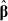i. This can be done by using least squares (linear or nonlinear, depending on the functional form of the degradation path).

 Solve the equation D(t;i;i)=Df for t and call the solutionl,...,n.

 Repeat the procedure for each sample path to obtain the pseudo failure times

l,...,n.

 Do a single distribution time-to-failure analysis (Nelson, 1990) of the data

l,...,n to estimate FT(t).

The approximate method is simple and intuitively appealing. However, it is only adequate in cases where the degradation path D(t) is relatively simple, the degradation model considered is sufficiently appropriate, there is enough degradation data to accurately estimate αi and βi, the magnitude of the errors is small and finally, the magnitude of the extrapolation needed to predict the failure times is small (Meeker & Escobar, 1998). Note that this method considers the model parameters as fixed.

Moreover, the approximate method presents the following problems: it ignores the errors in the prediction of the "pseudo" failure times

i and does not consider the errors involved in the observed degradation values, the distribution of the "pseudo" failure times does not generally correspond to the one that would be indicated by the degradation model and, in some cases, the volume of degradation data collected can be insufficient for estimating all the model parameters. In these scenarios, it might be necessary to fit different models for different units to predict the "pseudo" failure times.

3.1.2 The analytical method

For some simple path models, FT(t) can be expressed in a closed form. The following example provides an illustration of such a case.

Suppose that the actual degradation path of a particular unit is given by D(t)=α+βt, where α is fixed and represents the common initial amount of degradation of all the test units at the beginning of the test (D(0)=α), and therefore corresponds to a fixed effect; β is the degradation rate that varies from unit to unit and corresponds to a random effect.

Assuming that β varies from unit to unit according to a log-normal distribution with parameters μb and σb, it is possible to define the distribution function of T, by

where Φnor(.) is the cumulative distribution function of a standard normal distribution. In this case, T is also log-normal with location and scale parameters given by μT=[lod(Df-α)-υb] and σTb. Other probability density functions can be used along with the same procedures in order to obtain the failure time distribution FT(t). This method can be used for a simple nonlinear degradation model, assuming, for example, D(t)=αl+β exp(α2t) for β>0, where αl, α2 are fixed and β is a random effect. Results with other distributions like the Weibull, Normal (Gaussian) and the Bernstein distribution can be found in Lu & Meeker (1993).

3.1.3The two-stage method

To carry out the two-stage method of parameter estimation, the following steps should be implemented.

Stage 1

1. In the first stage, for each sampled unit i(i=1,2,...n), fit the degradation model (1) (using least squares) and obtain the Stage 1 estimates of the model parameters (α; βi). In other words, for each unit i, (i=1,2,...,n) (i,i) are the least squares estimators of the fixed and random model parameters. In addition, an estimator of the error variance, obtained from the ith unit is the mean square error2. Assume that, by some appropriate reparameterization (e.g., using a Box-Cox transformation)

i=H(i) is approximately multivariate normally distributed with the asymptotic mean μj and variance covariance matrix Σφ.

Stage 2

In the second stage, the unconditional estimators, from the preceding discussion,(

i,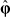i) (i=1,2,...n) are combined to construct the two-stage estimators of the path-model parameters. The two-stage estimators of the path-model parameters α,μφ and Σφ are, respectively:

Point estimation ofFT(t)

The estimate of

T(t) of FT(t) can be evaluated to any desired degree of precision by using Monte Carlo simulation. This is done by generating a sufficiently large number of random sample paths from the assumed path model with the estimated parameters and using the proportion failing as a function of time as an estimate of FT(t). The basic steps are:

1. Estimate the path-model parameters α,μφ and Σφ from the n sample paths by using the two-stage method, giving,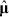φ and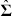φ.

2. Generate N simulated realizationsof φ from N(φ,φ) and obtain the corresponding N simulated realizations β* of β from H-1(

), where N is a large number (e.g., N=100,000) and H-1 is the inverse transformation of H. Note that in the cases where the distribution of Fβ(.) of β is known, N simulated realizations β* of β can be generated directly from this distribution. These values can then be used in the steps 3 and 4 described below.

3. Compute the corresponding N simulated failure times t* by substituting β* into Df=D(β;;t).

4. Estimate FT(t) from the simulated empirical distribution

for any desired values of t.

The Monte Carlo approximation error is easy to evaluate by using the binomial distribution. This error can be made arbitrarily small by choosing the Monte Carlo sample size N to be large enough. Pointwise confidence intervals can be constructed by the bootstrap procedures (Efron, 1985).

3.1.4 The numerical method

Many practical situations are described by nonlinear models which include more than one random effect. In these cases, it is very difficult to get a closed-form expression for FT(t). In such cases, estimation of the model parameters needs to be done by maximization of the likelihood function numerically.

Suppose that in the general degradation path model (1), the parameter vector Θt=(α;β)=(αl,...αpl,...βk) follows a Multivariate Normal Distribution (MVN), with mean vector μQ and variance-covariance matrix ΣΘ. In addition, suppose that the random errors {εij} follow a normal distribution with mean zero and constant variance. The assumption of MVN distribution for Θ allows the information of the unit path D(t) to be concentrated only on the parameters μΘ and ΣΘ without loss of information. For the fixed effects components of Θ, the values are set equal to the proper effects and the respective variance and covariance terms involving the fixed effects are set equal to zero.

The estimation of μΘΘ andis carried out from the following likelihood function:

whereand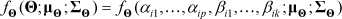is the multivariate normal density function.

Pinheiro & Bates (1995) used the results developed by Lindstrom & Bates (1990) to obtain the approximation maximum likelihood estimate of the parameters μΘΘ and. The LME (linear mixed effects models) and NLME (nonlinear mixed effects models) functions, written in the S-PLUS language, were developed to attain this goal (Pinheiro & Bates, 2000).

After the estimation of μΘΘ and, FT(t) can be obtained numerically by direct integration. The amount of computational time needed to evaluate the multidimensional integral will, however increase exponentially with the dimension of the integral. An alternative procedure is to evaluate FT(t) numerically using Monte Carlo Simulation.

This simulation is carried out using the estimates of the parameters μΘΘ and, that are supplied by the LME or NLME functions. N possible degradation paths D(t) are generated and for each one of them the "failure time" (crossing time or equivalently, the time when the degradation path first crosses the line y=Df) is obtained to calculate the values ofT(t) using the expression

where t is a fixed instant of time and N must be a large number (usually, N>105).

To simulate the N paths of D(t) it is necessary to generate N possible realizations of the vector Θt=(α;β)=(αl,...αpl,...βk) from a MVN distribution with meanΘ and variance-covariance matrixΘ. The last step consists of applying (3). An algorithm showing the whole sequence of estimations steps for the numerical method was presented by Yacout et al. (1996). Confidence intervals can be obtained using a resample method, as the Bootstrap (Efron, 1985).

3.2 Bayesian Inference

Consider the general degradation path model given by expression (1). For that model the βi´s(i=1,2,...,n) are assumed to be independently distributed as Λβ(θ), where Λβ(θ) is a multivariate distribution function, which may depend on an unknown parameter vector θ=(0l,...,0q)t that must be estimated from the data. In addition, {εij} and {βi} are assumed independent and the random errors εij´s (i=l,...n;j=1,...,mi) are assumed to be independently and identically distributed (iid) with mean zero and unknown variance. Under this degradation model, one has to get the estimates of the unknown model parameters η=(α;θ;)in order to estimate the failure time distribution.

Bayesian inference provides a way to estimate the unknown model parameters and to assess their uncertainty through the resulting parameter posterior distribution. It does so by combining prior information about η=(α;θ;) with the information about η=(α;θ;) contained in the data. The prior information is described by a probability density function π(η) known as the prior, and the information provided by the data is captured by the data sampling model l(Data|η)=l(y|η)=l(y11,...ylml,...,ynl,...ynmn|η), known as the likelihood. The combined information is then described by another probability density function π(η|ψ) called the posterior. Bayes theorem provides the way to calculate the posterior, namely,

where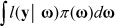is the marginal density of y.

The problem here is usually to calculate the integral in equation (4) as well as those necessary to get marginal posteriors from π(η|y). However recent advances in Bayesian computing make it easy to sample from the posterior of the model parameters (Geman & Geman, 1984; Gelfand & Smith, 1990; Casella & George, 1992; Chib & Greenberg, 1995). The sampling is accomplished through Markov Chain Monte Carlo (MCMC) simulation (Lopes & Gamerman, 2006). It also turns out that it is more convenient to work with samples to provide inference for the reliability function. For the wheel degradation data, the Bayesian software WinBugs (Spiegelhalter et al., 2000) was used to carry out Bayesian inference. WinBugs is freely available from the Web at http://www.mrc-bsu.cam.ac.uk/bugs/ and can easily implement MCMC.

3.2.1 Point Estimates

Although the posterior distribution π(η|y) summarizes all the information about η once the data y is observed, in some cases it is convenient to summarize this information in a single quantity. In a Bayesian framework it is necessary to first specify the losses consequent on making a decision d when various values of the parameter η pertain.

For a real valued parameter η and a loss function L(η,d), the Bayes estimator is the value d which minimizes the posterior expected loss. In other words,

Different loss functions lead to different Bayes estimators. If the quadratic loss function L(η,d)=(η-d)2 is used then d=B=E(η|y) (the posterior mean). It can be shown that the choices L(η,d)=|η-d| and the "0-1" loss generate respectively the posterior median and the posterior mode as Bayesian estimators (Migon & Gamerman, 1999). More general results are available. For instance, if the quadratic loss L(η,d)=(η-d)tM(η and d are now vectors and M is a positive definite matrix) is chosen, it can be shown that the Bayes estimator is still the posterior mean.

In addition to point summaries, it is always important to report posterior uncertainty. The usual approach it to present quantiles of the posterior distribution of the quantities of interest. A slightly different method of summarizing posterior uncertainty is to compute a region of highest posterior density (HPD): the region of values that contains 100(1-α) % of the posterior probability and also has the characteristic that the density within the region is never lower than that outside.

High Posterior Density regions (HPD) were calculated using the package Coda (Plummer et al., 2005) implemented in the software R (2006).

4. The Wheel Degradation Data revisited

The statistical model for the data displayed in Figure 1 can be succinctly stated as

where the reciprocal slope β is the ith unit random effect and represents an individual unit's characteristics (variations in the properties of the raw material, in the production process, in the component dimensions, etc.) and εij is the associated random error of the ith unit at time tij. It is assumed that 1) βi´s and the εij´s are independent and 2) εij are independently and identically distributed N(0,).

In this section, the wheel degradation data is analyzed. Sections 4.1 to 4.4 describe de data analysis based on each one of the "classical" methods. All the results are summarized in Table 2. Figures 4 to 6 summarize them graphically, including the confidence intervals that have been constructed in each case. Comments regarding the comparison of these results are left to Section 4.5. The Bayesian approach to this practical situation is described in Section 4.6.

4.1 Estimation ofFT(t) using the approximate method.

A separate degradation model given by the expression (6) was fitted to each sample unit i and least squares estimatorsi of βi (i=1,2,...,14) where calculated. Note that, by doing this, the model parameter βi is assumed to be fixed. The calculation of the "pseudo" failure distance for each wheel unit was carried out from the values ofi, using the expression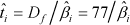. The results of this step are displayed in Table 1.

Probability plots and residual analysis were used to investigate the adequacy of several distributions to the data displayed in Table 1 (pseudo failure distances). Figure 2 shows probability plots for the Weibull and log-normal distributions. Figure 3 compares the Kaplan Meier nonparametric point estimates (km(t)) of the reliability function RT(t) (Meeker & Escobar, 1998) and the maximum likelihood (ML) estimatesW(t) andLN(t) obtained from the Weibull and log-normal models respectively, all evaluated at the pseudo failure times (pseudo failure distances) shown in Table 1.

Some observations from Figures 2 and 3 are:

 Figure 2 shows that either the log-normal or Weibull distribution can be used to fit the data displayed in Table 1

 Comparing Figures 3(a) and 3(b) shows that the points on Figures 3 (b) (log-normal) lie closer to the line "y=x" than the points on the Weibull plot. This pattern indicates that the parametric point estimates provided by the log-normal model are closer to the empirical estimates than the ones provided by the Weibull model, indicating a slightly better performance of the former. However, due to sample size restrictions (only 14 wheels), it was decided to go on the analysis considering both distributions.

Table 2 shows the results obtained from the Weibull and log-normal models. The estimated values of the average distance covered by the wheel (MTTF) are 1,060,880 Km (Weibull model) and 1,071,870 Km (log-normal model). Other quantities of interest are the median distance (t0.50), the 10th and the reliability at 300,000 Km. Table 2 displays these estimates as well as the 95% asymptotic confidence intervals. We note that the widths of the confidence intervals provided by the log-normal model are smaller than the respective ones calculated by the Weibull model, indicating a higher precision of the estimated values provided by the former.

4.2 Estimation of FT(t) using the analytical method

1. For each sampled unit, the degradation model (6) was fitted to the sample paths and the estimates of the model parameters were obtained using the least squares estimation method. These estimated values () are exactly the ones that have already been shown in Table 1 (Section 4.1).

2. The degradation model (6) postulated for the wheel profiles is very simple since it is a straight line with one random parameter only. In addition, the analysis of probability plots constructed to the

i (they are not shown here) indicated that either the log-normal or Weibull distribution could be used to fit those values. Therefore, the failure time distribution FT(t) can be obtained directly, using the following relationships:where

Consequently, the data analysis was carried on according to the following steps:

1. Log-normal and Weibull models were fitted to the

i values and the maximum likelihood estimatesβ,β (log-normal case) andβ,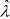β(Weibull case) were calculated.

2. Next, the parameters of the failure time distribution were obtained by using the expressions (7) and (8) above. The results are summarized below.

 Log-normal case:

β~log-normal (2.46192;0.644248)

T~log-normal([log77+2.46192];0.644248)

T~log-normal(6.805725; 0.644248)

 Weibull case:

β~Weibull (1.97619;15.54349)

T~Weibull(1.96719;77x15.54349)

T~Weibull(1.976719;1196.84873)

Table 2 summarizes the results based on the two distributions. 95% bootstrap confidence intervals were obtained for each one of the quantities of interest. For almost all of them it would have been possible to calculate asymptotic confidence intervals using the delta method (Mood, Graybill & Boes, 1974; chapt. 5, p. 181). One exception is the MTTF for which the calculations are not straightforward. Therefore, it was decided to calculate all the confidence intervals using the bootstrap (nonparametric) re-sampling method.

4.3 Estimation ofFT(t) using the two-stage

The steps of the analysis are given bellow.

1. As it was done in the approximate and the analytical method, for each sampled unit, the degradation model (6) was fitted to the sample paths and the estimates of the model parameters were obtained using the least squares estimation method (the estimated values

i are shown in Table 1).

2. Next, in order to use the two-stage estimation method, one would have to find an appropriate transformation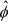=H(i), withi approximately normally distributed with asymptotic mean μf and asymptotic variance covariance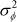. However as it was mentioned before, the analysis of probability plots constructed to thei´s indicated that either log-normal or a Weibull distribution could be used to fit those values, in particular, a log-normal(2.46192;0.644248) or a Weibull(1.976719;15.54349) (see results of the analytical method). Therefore, it was possible to move on to step 3, using these two distributions.

3. N=100,000 simulated realizations β* of β were generated from each one of the two distributions mentioned in step 2.

4. For each distribution, the corresponding N simulated failure times t* were calculated by substituting each β* into Df=βt.

5. FT(t) was estimated from the simulated empirical distribution

for any desired values of t.

Bootstrap 95% confidence intervals were calculated. The results are shown in Table 2.

4.4 Estimation ofFT(t) using the numerical method

The numerical method was not applied to this problem since there were evidences against the basic assumption regarding normality of the random parameter. Toledo (2007) showed that the results of the numerical method are strongly affected by the violation of that assumption.

4.5 Comparison of the results generated by the methods based on "classical" inference

Some observations from Table 2 and Figures 4 to 6 are:

1. The point estimates obtained by the Approximate and the Analytical methods are very similar. This result was already expected since there is a relationship between the random parameter distribution (Fβ) and the pseudo failure time distribution (FT).

2. The precision of the methods may be evaluated by the confidence intervals widths. These values are essentially the same for the central measures (MTTF and t0,50) for both distributions. Some differences can be detected for t0,10 and R (300,000). For t0,10, it seems that the two-stages method is slightly better than the other two, for the Weibull distribution. On the other hand, the Approximate method is the best one in the log-normal case. In terms of the R(300,000), the Approximate is the worst method for the two distributions considered.

3. Table 2 shows also the Akaike's Information Criterion - AIC (Akaike, 1974) calculated for the models based on the Weibull and log-normal distributions. Lower values of this index indicate the preferred model. For the approximate method for example, the values of this criterion are 219.062 and 219.30 for the Weibull and log-normal respectively. The same situation is observed for the analytical and the two-stages method, in other words, the AIC values for the Weibull-based model is slightly lower than the one for the log-normal. Note that the AIC values are the same for the analytical and the two-stages methods since in either case, the two distributions considered (Weibull and log-normal) are fitted to the same least squares estimates. Although the AIC values for Weibull-based models turned out to be smaller than the ones for the log-normal models, the observed difference is too small in order to be used as a model selection. Therefore, it is fair to say that for the situation studied, either the Weibull or the log-normal-based models can be used.

4.6 Bayesian Inference

In Section 4.1, pseudo lifetimes (obtained by fitting lines to each degradation curve and calculating the times when the fitted lines reach the failure threshold) were used to identify an appropriate lifetime distribution. The analysis showed that the pseudo lifetimes for the wheel degradation data are well described either by a Weibull or a log-normal distribution. In addition, the expressions (7) and (8) established the relationships between the reciprocal slopes {βi} and lifetimes distributions.

Consequently, in order to analyze the wheel degradation data, the following two models were considered.

Model 1: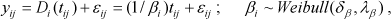therefore,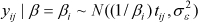for i=l,...,14 e j=1,...,12. In this case, the following flat priors were used:

δb~ Gamma(0.01;0.01),

λb ~Gamma(0.01;0.01) and~Inverse Gamma (0.01;0.01)

Gamma priors were chosen for δb and λb because they are positive quantities. The measurement error varianceis also a positive quantity, but there has been a tradition in the Bayesian literature to use an inverse gamma prior for this parameter (Migon & Gamerman, 1999). Consequently, the prior of the reciprocal ofis also a gamma distribution. The parameters δb, λb andare assumed independent.

Model 2:and the following priors:

μb~Normal(0;100),~Inverse Gamma (0.02; 0.02),~Inverse Gamma (0.01; 0.01)

These parameters are also assumed independent.

The priors adopted for models 1 and 2 are noninformative vagues (Migon & Gamerman, 1999) since they all have very large variances (flat densities). For instance, for model 1, the Gamma priors both have mean 1 and variance 100 and the Inverse Gamma has variance 1/0.01 = 100. Similarly, for model 2, the Inverse Gammas have both mean 1 and variances 200 and 100, forandrespectively. The Normal distribution adopted as prior is also very flat, with variance 100. These flat priors have been used in many practical situations found in the literature (Gelman, Carlin, Stern & Rubin, 2004).

The posterior of δb (shape parameter), λb (scale parameter),, βi (i=1,...14)(Weibull case) and of μb (location),(scale),, βi (i=1,...14) (log-normal case), were obtained by MCMC. A sample of size 102,000 was considered with a burn-in period of 2,000 draws and no thinning. The burn-in period was achieved by discarding the first 2,000 samples and because there was no thinning, the next 100,000 samples were kept. Convergence was assessed by graphical methods (Gamerman & Lopes, 2006). The results for the Weibull and log-normal cases are shown in Tables 3 and 4 respectively.

Note that for the Weibull case and the quadratic loss function, the reliability of the wheels at 300,000 Km is 0.92 (95% HPD region is [0.82;0.99]). In addition, 10% of the wheels will need replacement by 382.80 x 103 Km of usage (95% HPD: [151.86 103 Km; 611.34 103 Km]). The Bayesian estimates for the other quantities are given in Table 3 along with 95% HPD regions and selected quartiles of the posterior distribution.

In the log-normal case, the reliability of the wheels at 300,000 Km is 0.95 (95% HPD region: [0,86;1.00]). In addition, 10% of the wheels will need replacement by 405.60 103 Km of usage (95% HPD: [216.15 103 Km; 584.85 103 Km]). The Bayesian estimates for the other quantities are given in Table 4 along with 95% HPD regions and selected quantiles of the posterior distribution. The DIC value (Deviance Information Criterion; Spiegelhalter et al., 2002) for the Weibull and the log-normal models were 6309.27 and 6309.50, respectively indicating a similar performance of the two selected models.

Figures 7 and 8 show histograms of the posterior distributions of the MTTF (mean time to failure or mean covered distance); R(300,000) and t0,1 for the Weibull and lognormal models respectively. Note that R(300,000) has an asymmetrical distribution in both cases.

In this paper, five methods of degradation data analysis were presented. Four of them are based on the so called "classical" inference. The numerical method was not applied to the real data set since a basic model assumption was not valid in that situation. The point estimates obtained by each one of the "classical" methods were very similar. In particular, due to the relationship between the random parameter distribution and the failure time distribution, it was found that the Approximate and the Analytical methods lead to the same results. For more complicated models (nonlinear, more than one random parameter or even a mixed parameter model), the application of those methods might be difficult and may lead to different results. In these cases, researches will have to use the numerical method, assuming that the vector of random parameters has a multivariate normal distribution.

On the other hand Bayesian approach seems to be a reasonable choice especially if one needs to handle more complicated degradation models. Because reliability and lifetime distribution quantiles are functions of the model parameters, posteriors for these quantities are easily obtained from draws from the model parameter posteriors; for each such draw, simply evaluate the quantity of interest to obtain draws from that quantity's posterior.

One should be careful to compare the results of Bayesian and "classical" approaches since the concepts behind them are quite different. The former leads to a posterior distribution of the (random) quantity of interest while the latter produces a point estimate (of a fixed quantity). In "classical" approaches, confidence intervals are constructed while credible intervals are obtained in the Bayesian methods. But in practical situations like the one described in this paper, it is necessary to report some kind of "point estimate" in order to support future technical decisions. In that case, it is fair to say that by using flat priors and the quadratic loss function, Bayesian and classical approaches leaded roughly to the same results.

Acknowledgements

The authors are grateful to Fapemig (Fundação de Amparo à Pesquisa de Minas Gerais) and CNPq (Conselho Nacional de Pesquisa) for the support of this research. Also, our sincere gratitude is extended to the anonymous referees and the associate editor whose criticism led to a substantially improved paper.

(18) Minitab. Statistical Package, release 15.

Recebido em 07/2008; aceito em 07/2009

Received July 2008; accepted July 2009

• (1) Akaike, H. (1974). A new look at the statistical model identification. IEEE Transactions on Automatic Control, 19, 716-723.
• (2) Casella, G. & George, E.I. (1992). Explaining the gibbs sampler. The American Statistician, 46(3), 167-174.
• (3) Chib, S. & Greenberg, E. (1995). Understanding the Metropolis-Hasting Algorithm. The American Statistician, 49(4), 327-335.
• (4) Crk, V. (2000). Reliability Assessment from Degradation Data. Proceedings of the Annual Reliability and Maintainability Symposium, 155-161.
• (5) Doksum, K.A. (1991). Degradation Rate Models for Failure Time and Survival Data. CWI Quart, 4, 195-203.
• (6) Efron, B. (1985). Bootstrap Confidence Intervals for a Class of Parametric Problems. Biometrika, 72, 45-58.
• (7) Gamerman, D. & Lopes, H.F. (2006). Markov Chain Monte Carlo Stochastic simulation for Bayesian inference (2nd edition). Texts in Statistical Science. Chapman & Hall.
• (8) Gelfand, A.E. & Smith, A.F.M. (1990). Sampling-based approaches to calculating marginal densities. Journal of the American Statistical Association, 85, 398-409.
• (9) Geman, S. & Geman, D. (1984). Stochastic relaxation, Gibbs distributions and the Bayesian restoration of images. IEEE Transactions on Pattern Analysis and Machine Intelligence, 6, 721-741.
• (10) Gertsbackh, I.B. & Kordonskiy, K.B. (1969). Models of Failure Springer-Verlag, New York.
• (11) Hamada, M. (2005). Using Degradation Data to Assess Reliability. Quality Engineering, 17, 615-620.
• (12) Jiang, M. & Zhang, Y. (2002). Dynamic Modeling of Degradation Data. Proceedings of the Annual Reliability and Maintainability Symposium, 607-611.
• (13) Lindstrom, M.J. & Bates, D.M. (1990). Nonlinear Mixed Effects Models for Repeated Measures Data. Biometrics, 46, 673-687.
• (14) Lu, C.J. & Meeker, W.Q. (1993). Using Degradation Measures to Estimate a Time-to-Failure Distribution. Technometrics, 35, 161-174.
• (15) Marseguerra, M.; Zio, E. & Cipollone, M. (2003). Designing Optimal Degradation Tests Via Multi-Objective Genetic Algorithms. Reliability Engineering & System Safety, 79, 287-294.
• (16) Meeker, W.Q. & Escobar, L.A. (1998). Statistical Methods for Reliability Data Wiley, New York.
• (17) Migon, H.S. & Gamerman, D. (1999). Statistical Inference: An Integrated Approach Arnold, Londres.
• (19) Mood, A.A.; Graybill, F.A. & Boes, D.C. (1974). Introduction to the Theory of Statistics 3rd edition. McGraw Hill, London.
• (20) Nelson, W. (1981). Analysis of Performance Degradation Data from Accelerated Tests. IEEE Transactions on Reliability, 30, 149-155.
• (21) Nelson, W. (1990). Accelerated Testing: Statistical Methods, Test Plans, and Data Analysis Wiley, New York.
• (22) Pinheiro, J.C. & Bates D.C. (1995). Approximations to the log-likelihood function in The nonlinear mixed-effects model. Journal of Computational and Graphical Statistics, 1(4), 12-35.
• (23) Pinheiro, J.C. & Bates D.C. (2000). Mixed-Effects Models in S and S-PLUS Springer-Verlag, New York.
• (24) Plummer, M.; Best, N.; Cowles, K. & Vines, K. (2005). Output analysis and diagnostics for MCMC The Coda Package. Software R. Available at <http://www.fis.iarc.fr/coda/>
• (25) R Development Core Team (2006). R: A language and environment for statistical computing. R Foundation for Statistical Computing, Vienna, Austria. ISBN 3-900051-07-0, URL <http://www.R-project.org>
• (26) Spiegelhalter, D.J.; Best, N.G.; Carlin, B.P. & van der Linde, A. (2002). Bayesian measures of model complexity and fit (with discussion and rejoinder). Journal of the Royal Statistical Society, Series B, 64, 583-639.
• (27) Spiegelhalter, D.J.; Thomas, A. & Best, N. (2000). WinBUGS v.1.4 User Manual available at <http://www.mrc-bsu.cam.ac.uk/bugs/>
• (28) Su, C.; Lu, J.C.; Chen, D. & Hughes-Oliver, J.M.A. (1999). Random Coefficient Degradation Model with Random Sample Size. Lifetime Data Analysis, 5, 173-183.
• (29) Toledo, M.L.G. de (2007). Ensaios de Degradação: Estudo Comparativo de Métodos de Análise. Dissertação de Mestrado. Departamento de Estatística, Universidade Federal de Minas Gerais.
• (30) Tseng, S.T.; Hamada, M. & Chiao, C.H. (1995). Using Degradation Data to Improve Fluorescent Lamp Reliability. Journal of Quality Technology, 27, 363-369.
• (31) Whitmore, G.A. & Shenkelberg, F. (1997). Modelling accelerated degradation data using Wiener diffusion with a time scale transformation. Lifetime Data Analysis, 3, 27-45.
• (32) Wu, S.J. & Chang, C.T. (2002). Optimal Design of Degradation Tests in Presence of Cost Constraint. Reliability Engineering & System Safety, 76, 109-115.
• (33) Wu, S.J. & Shao, J. (1999). Reliability Analysis Using the Least Squares Method in Nonlinear Mixed-Effect Degradation Models. Statist Sinica, 9, 855-877.
• (34) Wu, S.J. & Tsai, T.R. (2000). Estimation of Time-to-Failure Distribution Derived From a Degradation Model Using Fuzzy Clustering. Quality and Reliability Engineering International, 16, 261-267.
• (35) Yacout, A.M.; Salvatores, S. & Orechwa, Y. (1996). Degradation Analysis Estimates of the Time-to-Failure Distribution of Irradiated Fuel Elements. Nuclear Technology, 113, 177-189.
• (36) Yu, H.F. & Tseng, S.T. (1997). Designing a Degradation Experiment. Naval Research Logistics, 46, 689-706.
• (37) Yu, H.F. & Chiao, C.H. (2002). An Optimal Designed Degradation Experiment for Reliability Improvement. IEEE Trans on Reliability, 51(4), 427-433.
• (38) Yu, H.F. & Tseng, S.T. (2004). Designing a Degradation Experiment with a Reciprocal Weibull Degradation Rate. Quality Technology and Quantitative Management, 1(1), 47-63.
• *
Corresponding author / autor para quem as correspondências devem ser encaminhadas
• # Publication Dates

• Publication in this collection
27 May 2010
• Date of issue
Apr 2010